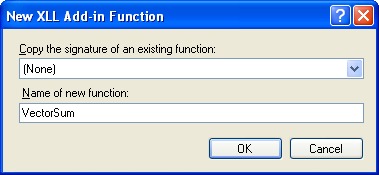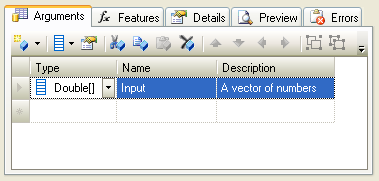XLL+ Class Library (7.0)

# Adding a vector argument

You can use the XLL+ Function Wizard to specify that an argument should contain a vector of a particular type, e.g. a row of strings or a column of dates. This walkthrough demonstrates how to add and use a vector argument in an add-in function.

You can find a copy of all the code used in this walkthrough in the `Walkthroughs/VectorFns` folder in your XLL+ installation, along with a demonstration spreadsheet, `VectorFns.xls`.

### Creating the project

#### To create the project using Visual Studio

1. From the File menu, select New and then Project to open the New Project dialog.
2. Select the XLL+ 7 Excel Add-in project template from the list of Visual C++ Projects, and enter VectorFns in the Name box. Under Location, enter an appropriate directory in which to create the project.
3. Accept all the default settings in the XLL+ AppWizard.

For more information about creating projects, see Creating an add-in project in the XLL+ User Guide.

### Creating the function

Use the XLL+ Function Wizard to create a new add-in function and to add a vector argument.

Note: If you do not know how to start the Function Wizard, or you cannot find the command to open it, look at Invoke the Function Wizard in the User Guide.

1. Open the source file VectorFns.cpp and click on the New XLL+ Function menu item or tool-button, to show the New Function dialog, and fill in the name as shown below.Name VectorSum CXlOper Math & Trig Returns the sum of a vector
2. Fill in the function's category and description.

Add a new argument named Input of type Double, by typing into the arguments grid, as shown below.3. Click on the Vector tool, to convert the argument to a vector.4. The argument is now a vector of type double, as shown below.Type Name Description
Double[] Input A vector of numbers

Click on the OK button to close the Function Wizard and save the function.

### Inspecting the code

1. The following code has been added to VectorFns.cpp.CopyC++
```CXlOper* VectorSum_Impl(CXlOper& xloResult, const CXlOper* Input_op)
{
// Input buffers
std::vector<double> Input;
// Validate and translate inputs
XlReadVector(*Input_op, Input, L"Input", XLA_TRUNC_ONEMPTY|XLA_TRUNC_ONBLANK);
// End of generated code
//}}XLP_SRC
// TODO - set the value of xloResult, or return another value
//          using CXlOper::RetXXX() or throw a CXlRuntimeException.
return xloResult.Ret();
}```

Let us examine the interesting code.

2. Note that the argument has been declared as type const CXlOper*. The CXlOper type is usually used to pass vector and matrix values from Excel to add-in functions.CopyC++
`CXlOper* VectorSum_Impl(CXlOper& xloResult, const CXlOper* Input_op)`
3. A buffer variable Input is declared. The contents of the COper argument passed by Excel will be extracted and put into Input.CopyC++
`std::vector<double> Input;`
4. Code has been generated to read the contents of Input into vecInput.CopyC++
`XlReadVector(*Input_op, Input, L"Input", XLA_TRUNC_ONEMPTY|XLA_TRUNC_ONBLANK);`

Note that if the cell range passed to the function cannot be read for any reason (for example, because a cell contains a string instead of a number) then an exception will be thrown, and no further processing will take place within the function. Instead, control will pass to an outer wrapper function, which will catch the exception, and return it to Excel as an error result.

### Completing the function

1. Add code to implement the function, as shown below:CopyC++
```CXlOper* VectorSum_Impl(CXlOper& xloResult, const CXlOper* Input_op)
{
// Input buffers
std::vector<double> Input;
// Validate and translate inputs
XlReadVector(*Input_op, Input, L"Input", XLA_TRUNC_ONEMPTY|XLA_TRUNC_ONBLANK);
// End of generated code
//}}XLP_SRC

double dSum = 0.0;
for (size_t i = 0; i < Input.size(); i++)
dSum += Input[i];
xloResult = dSum;
return xloResult.Ret();
}```

### Testing the project

1. Build the project, using the Build/Build Solution menu or Shift+Ctrl+B keyboard short-cut. If you are working with a 64-bit version of Excel, then be sure to select the 64-bit platform `x64` before you build.
2. When the project has been built successfully, use the Debug/Start Debugging menu or F5 keyboard short-cut to run your add-in under Excel.
3. If Excel puts up any message boxes asking whether you trust the add-in, say yes.
4. In a spreadsheet, put numbers into cells A1 to A6 and, in cell B1 enter a formula `=VectorSum(A1:A6)` to return the sum of the numbers in cells A1 to A6.
5. Replace one of the numbers in cells A1:A6 with a non-numeric, e.g.: `Fred`. Note that the result is now an error message, such as: `#ERROR: Expected number for Input`